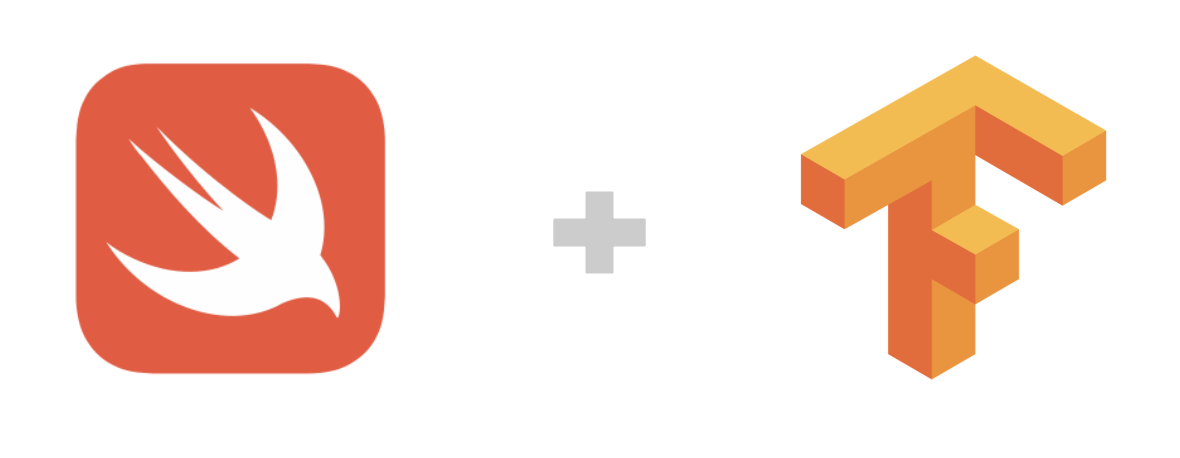# Swift for TensorFlow (S4TF) (Huan）¶“Swift for TensorFlow is an attempt to change the default tools used by the entire machine learning and data science ecosystem.”

– Jameson Toole, Co-founder & CTO of Fritz.ai

## S4TF 简介¶

Google 推出的 Swift for TensorFlow （简称S4TF）是专门针对 TensorFlow 优化过的 Swift 版本。（目前处在 Pre-Alpha 阶段）

Swift 语言创始人 Chris Lattner

Swift 语言是 Chris Lattner 在苹果公司工作时创建的。 现在 Chris Lattner 在 Google Brain 工作，专门从事深度学习的研究，并为 Swift 重写了编译器，为 TensorFlow 做定制优化。

### 为什么要使用 Swift 进行 TensorFlow 开发¶

1. 开发效率高：强类型语言，能够静态检查变量类型

2. 迁移成本低：与 Python，C，C++ 能够无缝结合

3. 执行性能高：能够直接编译为底层硬件代码

4. 专门为机器学习打造：语言原生支持自动微分系统

## S4TF 环境配置¶

### 在 Colaboratory 中快速体验 Swift for TensorFlow¶

Google 的 Colaboratory 可以直接支持 Swift 语言的运行环境。可以 点此 直接打开一个空白的，具备 Swift 运行环境的 Colab Notebook ，这是立即体验 Swift for TensorFlow 的最方便的办法。

### 在 Docker 中快速体验 Swift for TensorFlow¶

1. 获得一个 S4TS 的 Jupyter Notebook

在命令行中执行 `nvidia-docker run -ti --rm -p 8888:8888 --cap-add SYS_PTRACE -v "\$(pwd)":/notebooks zixia/swift` 来启动 Jupyter ，然后根据提示的 URL ，打开浏览器访问即可。

2. 执行一个本地的 Swift 代码文件

为了运行本地的 `s4tf.swift` 文件，我们可以用如下 docker 命令：

```nvidia-docker run -ti --rm --privileged --userns=host \
-v "\$(pwd)":/notebooks \
zixia/swift \
swift ./s4tf.swift
```

## S4TF 基础使用¶

Swift 是动态强类型语言，也就是说 Swift 支持通过编译器自动检测类型，同时要求变量的使用要严格符合定义，所有变量都必须先定义后使用。

```var n = 42
n = "string"
```

```Cannot assign value of type 'String' to type 'Int'
```

```import TensorFlow

// 声明两个Tensor
let x = Tensor<Float>()
let y = Tensor<Float>()

// 对两个 Tensor 做加法运算
let w = x + y

// 输出结果
print(w)
```

`Tensor<Float>` 中的 `<Float>`

### 在 Swift 中使用标准的 TensorFlow API¶

`import TensorFlow` 之后，既可以在 Swift 语言中，使用核心的 TensorFlow API。

1. 处理数字和矩阵的代码，API 与 TensorFlow 高度保持了一致：

```let x = Tensor<BFloat16>(zeros: [32, 128])
let h1 = sigmoid(matmul(x, w1) + b1)
let h2 = tanh(matmul(h1, w1) + b1)
let h3 = softmax(matmul(h2, w1) + b1)
```
1. 处理 Dataset 的代码，基本上将 Python API 中的 `tf.data.Dataset` 同名函数直接改写为 Swift 语法即可直接使用：

```let imageBatch = Dataset(elements: images)
let labelBatch = Dataset(elements: labels)
let zipped = zip(imageBatch, labelBatch).batched(8)

let imageBatch = Dataset(elements: images)
let labelBatch = Dataset(elements: labels)
for (image, label) in zip(imageBatch, labelBatch) {
let y = matmul(image, w) + b
let loss = (y - label).squared().mean()
print(loss)
}
```

`matmul()` 的别名： `•`

### 在 Swift 中直接加载 Python 语言库¶

Swift 语言支持直接加载 Python 函数库（比如 NumPy），也支持直接加载系统动态链接库，很方便的做到即导入即用。

```import Python

let np = Python.import("numpy")
let x = np.array([[1, 2], [3, 4]])
let y = np.array([11, 12])
print(x.dot(y))
```

```[35 81]
```

```import Glibc
let x = malloc(18)
memcpy(x, "memcpy from Glibc", 18)
free(x)
```

### 语言原生支持自动微分¶

```@differentiable
func frac(x: Double) -> Double {
return 1/x
}

```

```-4.0
```

Swift 函数声明中的参数名称和类型

Swift 使用 `func` 声明一个函数。在函数的参数中，变量名的冒号后面代表的是“参数类型”；在函数参数和函数体（`{}`） 之前，还可以通过瘦箭头（`->`）来指定函数的``返回值类型``。

### MNIST数字分类¶

1. 首先，引入S4TF模块 `TensorFlow`、Python桥接模块 `Python`，基础模块 `Foundation` 和 MNIST 数据集模块 `MNIST`

```import TensorFlow
import Python
import Foundation

import MNIST
```

Swift MNIST Dataset 模块

Swift MNIST Dataset 模块是一个简单易用的 MNIST 数据集加载模块，基于 Swift 语言，提供了完整的数据集加载 API。项目 Github：https://github.com/huan/swift-MNIST

1. 其次，声明一个最简单的 MLP 神经网络架构，将输入的 784 个图像数据，转换为 10 个神经元的输出：

```struct MLP: Layer {
// 定义模型的输入、输出数据类型
typealias Input = Tensor<Float>
typealias Output = Tensor<Float>

// 定义 flatten 层，将二维矩阵展开为一个一维数组
var flatten = Flatten<Float>()
// 定义全连接层，输入为 784 个神经元，输出为 10 个神经元
var dense = Dense<Float>(inputSize: 784, outputSize: 10)

@differentiable
public func callAsFunction(_ input: Input) -> Output {
var x = input
x = flatten(x)
x = dense(x)
return x
}
}
```

Swift 参数标签

Swift 中，每个函数参数都有一个 参数标签 (Argument Label) 以及一个 参数名称 (Parameter Name)。 参数标签 主要应用在调用函数的情况，使得函数的实参与真实命名相关联，更加容易理解实参的意义。同时因为有 参数标签 的存在，实在的顺序是可以随意改变的。

1. 接下来，我们实例化这个 MLP 神经网络模型，实例化 MNIST 数据集，并将其存入 `imageBatch``labelBatch` 变量：

```var model = MLP()
let optimizer = Adam(for: model)

let mnist = MNIST()
let ((trainImages, trainLabels), (testImages, testLabels)) = mnist.loadData()

let imageBatch = Dataset(elements: trainImages).batched(32)
let labelBatch = Dataset(elements: trainLabels).batched(32)
```
1. 然后，我们通过对数据集的循环，计算模型的梯度 `grads` 并通过 `optimizer.update()` 来反向传播更新模型的参数，进行训练：

```for (X, y) in zip(imageBatch, labelBatch) {
// 计算梯度
let grads = gradient(at: model) { model -> Tensor<Float> in
let logits = model(X)
return softmaxCrossEntropy(logits: logits, labels: y)
}

// 优化器根据梯度更新模型参数
}
```

Swift 闭包函数（Closure）

Swift 的闭包函数声明为：`{ (parameters) -> return type in statements }`，其中：`parameters` 为闭包接受的参数，`return type` 为闭包运行完毕的返回值类型，`statements` 为闭包内的运行代码。

Swift 尾随闭包语法 (Trailing Closure Syntax)

Swift 输入输出参数 (In-Out Parameters)

1. 最后，我们使用训练好的模型，在测试数据集上进行检查，得到模型的准度：

```let logits = model(testImages)
let acc = mnist.getAccuracy(y: testLabels, logits: logits)

print("Test Accuracy: \(acc)" )
```

```Downloading train-images-idx3-ubyte ...